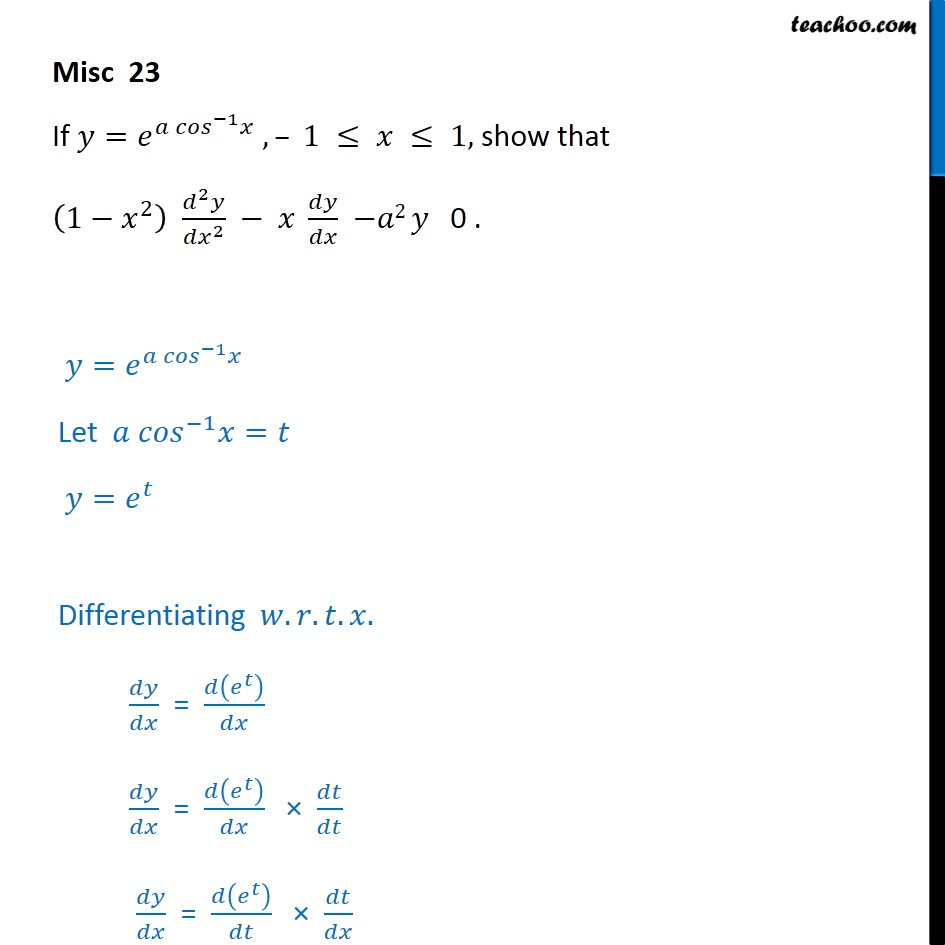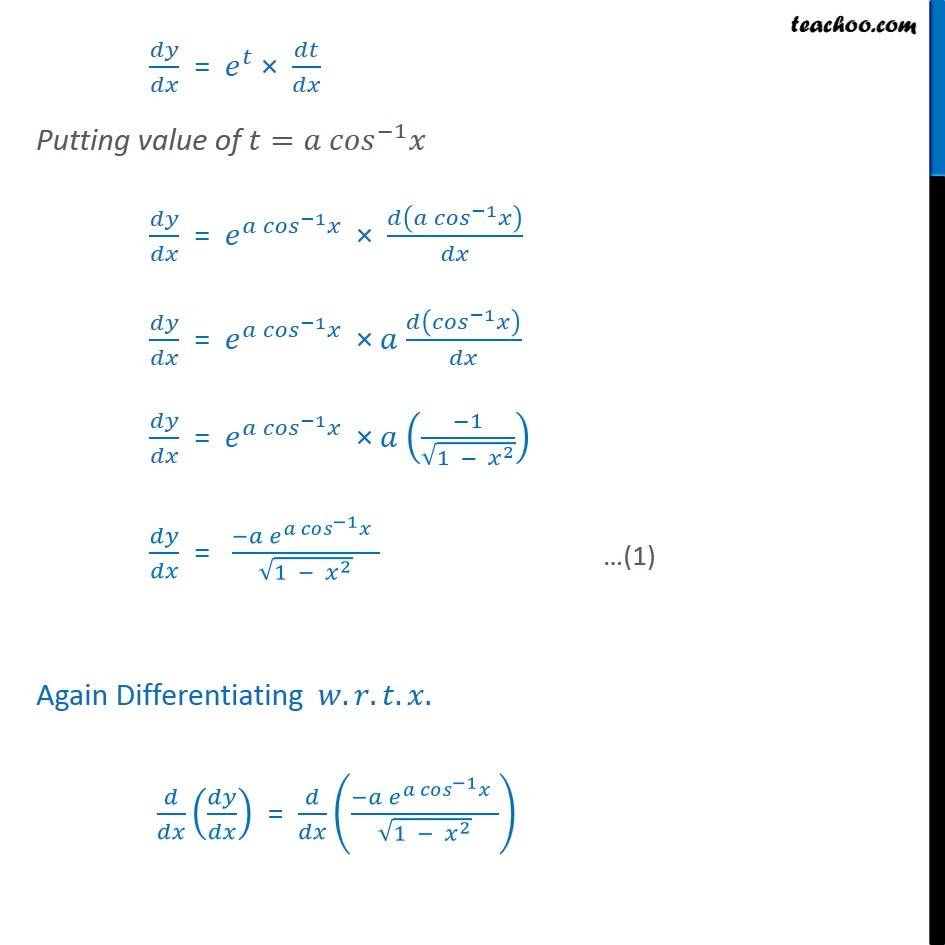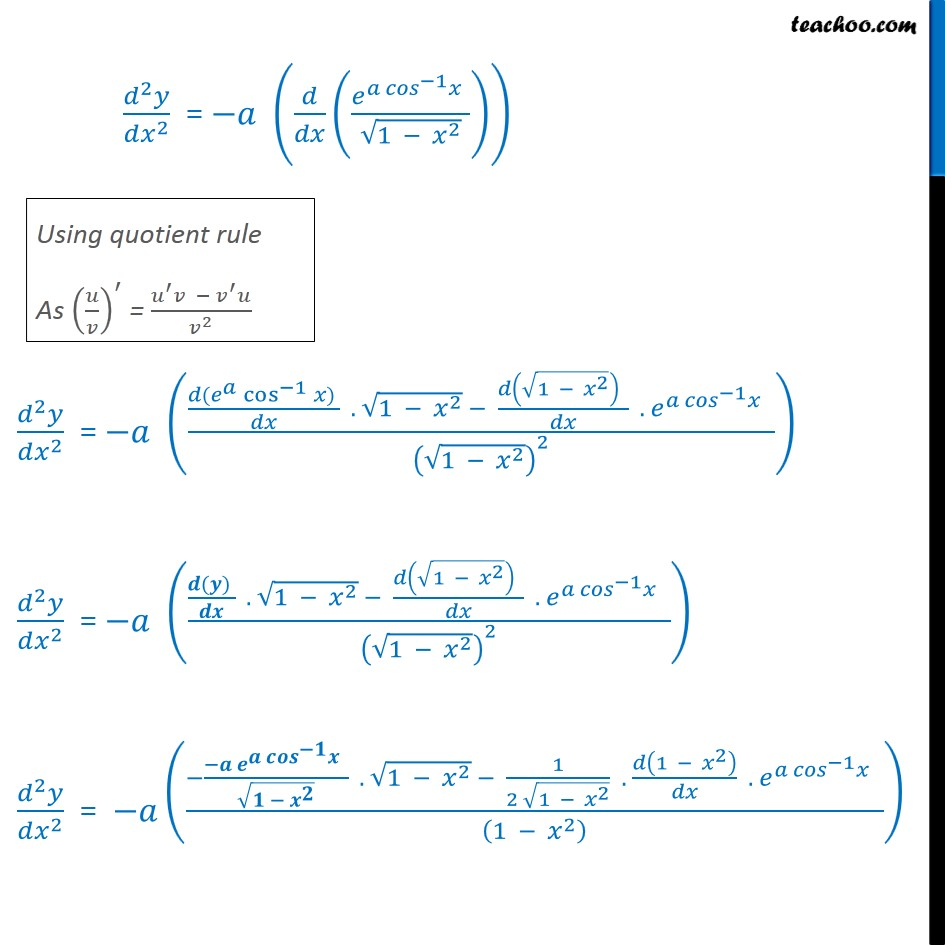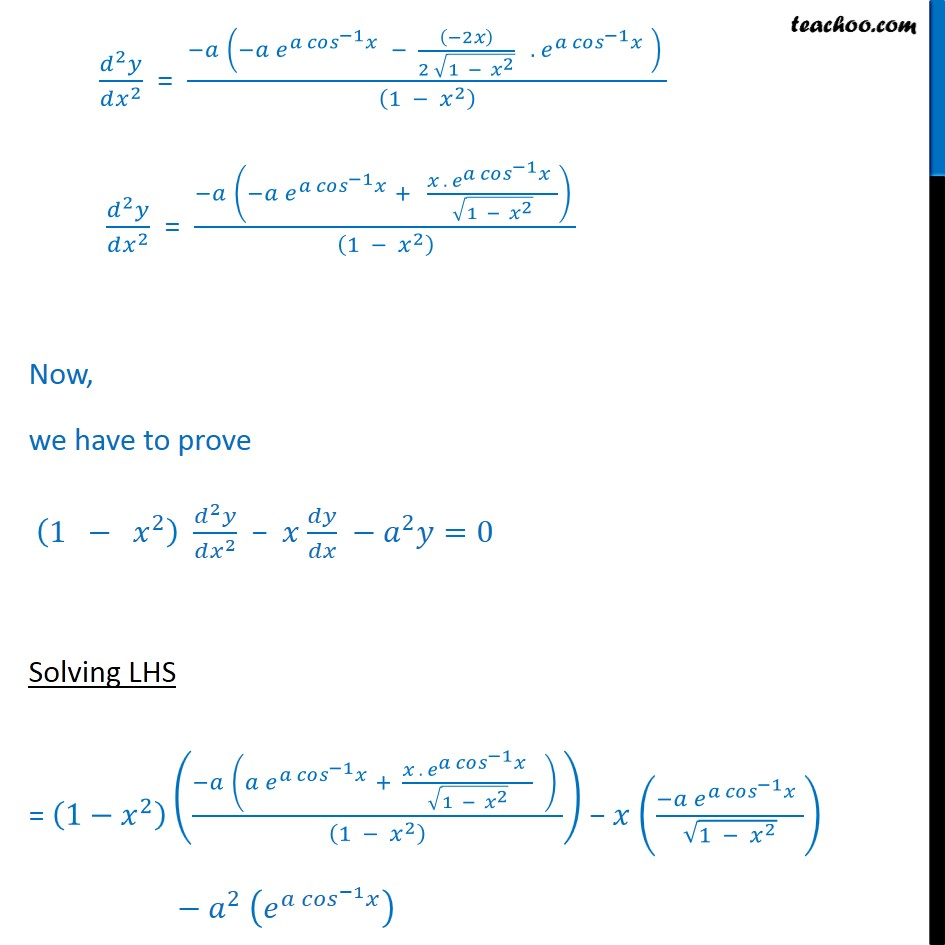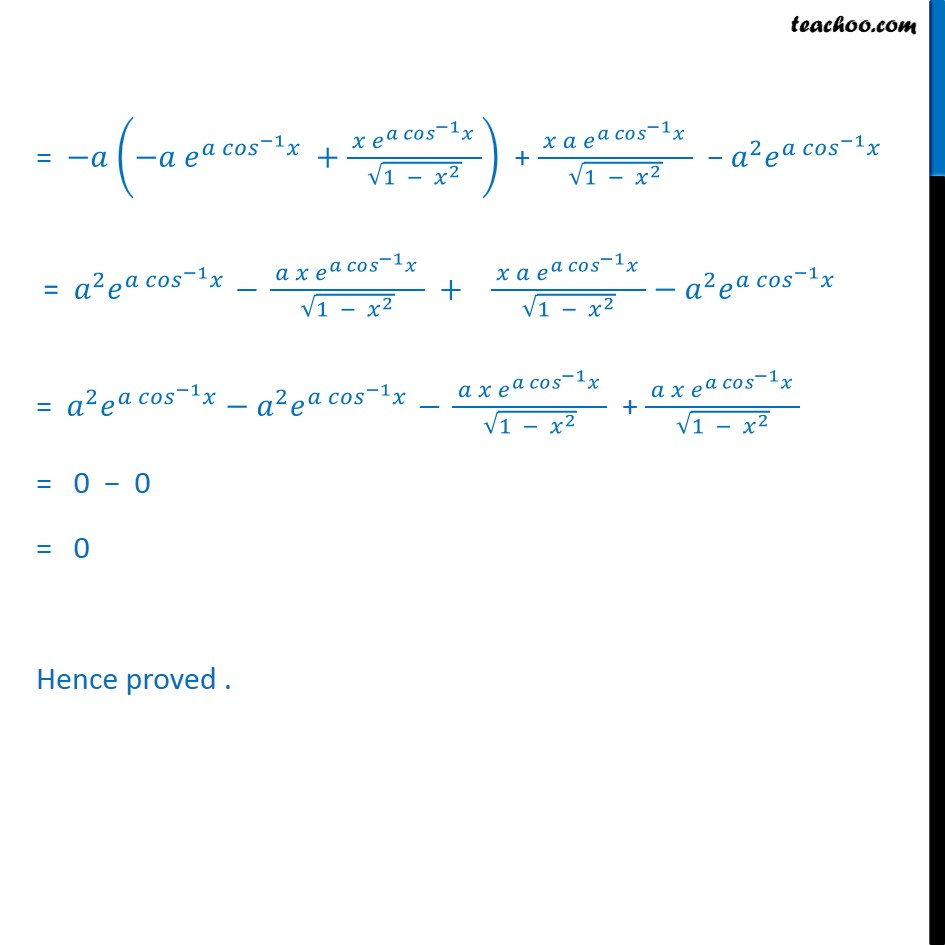1. Chapter 5 Class 12 Continuity and Differentiability
2. Serial order wise
3. Miscellaneous

Transcript

Misc 23 If 𝑦=𝑒^(〖𝑎 𝑐𝑜𝑠〗^(−1) 𝑥) , – 1 ≤ 𝑥 ≤ 1, show that (1−𝑥^2 ) (𝑑^2 𝑦)/〖𝑑𝑥〗^2 − 𝑥 𝑑𝑦/𝑑𝑥 −𝑎2 𝑦 0 . 𝑦=𝑒^(〖𝑎 𝑐𝑜𝑠〗^(−1) 𝑥) Let 〖𝑎 𝑐𝑜𝑠〗^(−1) 𝑥=𝑡 𝑦=𝑒^𝑡 Differentiating 𝑤.𝑟.𝑡.𝑥. 𝑑𝑦/𝑑𝑥 = 𝑑(𝑒^𝑡 )/𝑑𝑥 𝑑𝑦/𝑑𝑥 = 𝑑(𝑒^𝑡 )/𝑑𝑥 × 𝑑𝑡/𝑑𝑡 𝑑𝑦/𝑑𝑥 = 𝑑(𝑒^𝑡 )/𝑑𝑡 × 𝑑𝑡/𝑑𝑥 𝑑𝑦/𝑑𝑥 = 𝑒^𝑡 × 𝑑𝑡/𝑑𝑥 Putting value of 𝑡=〖𝑎 𝑐𝑜𝑠〗^(−1) 𝑥 𝑑𝑦/𝑑𝑥 = 𝑒^(〖𝑎 𝑐𝑜𝑠〗^(−1) 𝑥" " ) × 𝑑(〖𝑎 𝑐𝑜𝑠〗^(−1) 𝑥)/𝑑𝑥 𝑑𝑦/𝑑𝑥 = 𝑒^(〖𝑎 𝑐𝑜𝑠〗^(−1) 𝑥" " ) × 𝑎 𝑑(〖𝑐𝑜𝑠〗^(−1) 𝑥)/𝑑𝑥 𝑑𝑦/𝑑𝑥 = 𝑒^(〖𝑎 𝑐𝑜𝑠〗^(−1) 𝑥" " ) × 𝑎 ((−1)/√(1 − 𝑥^2 )) 𝑑𝑦/𝑑𝑥 = (−𝑎 𝑒^(〖𝑎 𝑐𝑜𝑠〗^(−1) 𝑥" " ))/√(1 − 𝑥^2 ) Again Differentiating 𝑤.𝑟.𝑡.𝑥. 𝑑/𝑑𝑥 (𝑑𝑦/𝑑𝑥) = 𝑑/𝑑𝑥 ((−𝑎 𝑒^(〖𝑎 𝑐𝑜𝑠〗^(−1) 𝑥" " ))/√(1 − 𝑥^2 ))

Miscellaneous